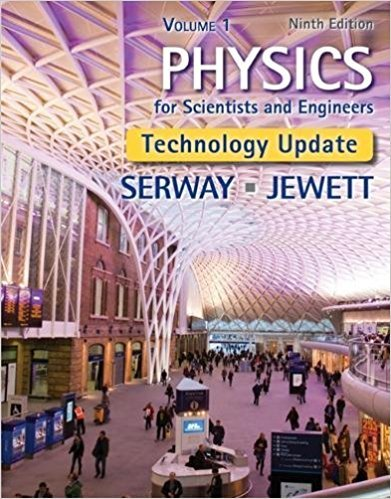×
Get Full Access to Physics For Scientists And Engineers, Volume 1, Technology Update - 9 Edition - Chapter 20 - Problem 79
Get Full Access to Physics For Scientists And Engineers, Volume 1, Technology Update - 9 Edition - Chapter 20 - Problem 79

×

# A cooking vessel on a slow burner contains 10.0 kg ofwater and an unknown mass of ice inISBN: 9781305116405 466

## Solution for problem 79 Chapter 20

Physics for Scientists and Engineers, Volume 1, Technology Update | 9th Edition

• Textbook Solutions
• 2901 Step-by-step solutions solved by professors and subject experts
• Get 24/7 help from StudySoup virtual teaching assistantsPhysics for Scientists and Engineers, Volume 1, Technology Update | 9th Edition

4 5 1 288 Reviews
23
0
Problem 79

A cooking vessel on a slow burner contains 10.0 kg ofwater and an unknown mass of ice in equilibrium at0C at time t 5 0. The temperature of the mixture ismeasured at various times, and the result is plotted inFigure P20.79. During the first 50.0 min, the mixtureremains at 0C. From 50.0 min to 60.0 min, the temperatureincreases to 2.00C. Ignoring the heat capacityof the vessel, determine the initial mass of the ice.012320 40 60T (C)t (min)0Figure P20.79

Step-by-Step Solution:
Step 1 of 3

Notes for Week 2 Physics 1 Instantaneous Velocity: Examples: 1. A jet engine moves along an experimental track (which we call the x axis). We will treat the engine as if it were a particle. Its position as a function of time is given by the 2 2 equation x = At + B, where A = 2.10m/s and B = 2.80m. (a) Determine the average velocity during this time interval. (b) Determine the displacement of the engine during the time interval from t1= 3.00s to t2= 5.00s. (c) Determine the magnitude of the instantaneous velocity at t = 5.00s. Solution: (a) In physics, the first thing you do when you have a question is see what the question is asking yo

Step 2 of 3

Step 3 of 3

##### ISBN: 9781305116405

The answer to “A cooking vessel on a slow burner contains 10.0 kg ofwater and an unknown mass of ice in equilibrium at0C at time t 5 0. The temperature of the mixture ismeasured at various times, and the result is plotted inFigure P20.79. During the first 50.0 min, the mixtureremains at 0C. From 50.0 min to 60.0 min, the temperatureincreases to 2.00C. Ignoring the heat capacityof the vessel, determine the initial mass of the ice.012320 40 60T (C)t (min)0Figure P20.79” is broken down into a number of easy to follow steps, and 78 words. Since the solution to 79 from 20 chapter was answered, more than 320 students have viewed the full step-by-step answer. This full solution covers the following key subjects: . This expansive textbook survival guide covers 22 chapters, and 1765 solutions. Physics for Scientists and Engineers, Volume 1, Technology Update was written by and is associated to the ISBN: 9781305116405. The full step-by-step solution to problem: 79 from chapter: 20 was answered by , our top Physics solution expert on 03/16/18, 04:11PM. This textbook survival guide was created for the textbook: Physics for Scientists and Engineers, Volume 1, Technology Update, edition: 9.

Unlock Textbook Solution